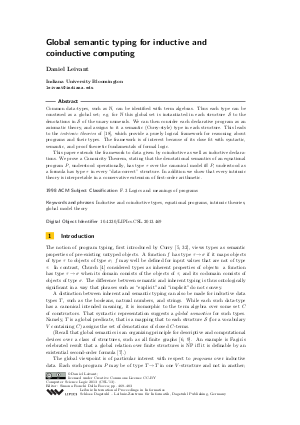Document# Global semantic typing for inductive and coinductive computing

### Author Daniel Leivant## File

LIPIcs.CSL.2013.469.pdf
• Filesize: 495 kB
• 15 pages

## Cite As

Daniel Leivant. Global semantic typing for inductive and coinductive computing. In Computer Science Logic 2013 (CSL 2013). Leibniz International Proceedings in Informatics (LIPIcs), Volume 23, pp. 469-483, Schloss Dagstuhl - Leibniz-Zentrum für Informatik (2013)
https://doi.org/10.4230/LIPIcs.CSL.2013.469

## Abstract

Common data-types, such as N, can be identified with term algebras. Thus each type can be construed as a global set; e.g. for N this global set is instantiated in each structure S to the denotations in S of the unary numerals. We can then consider each declarative program as an axiomatic theory, and assigns to it a semantic (Curry-style) type in each structure. This leads to the intrinsic theories of [Leivant, 2002], which provide a purely logical framework for reasoning about programs and their types. The framework is of interest because of its close fit with syntactic, semantic, and proof theoretic fundamentals of formal logic. This paper extends the framework to data given by coinductive as well as inductive declarations. We prove a Canonicity Theorem, stating that the denotational semantics of an equational program P, understood operationally, has type \tau over the canonical model iff P, understood as a formula has type \tau in every "data-correct" structure. In addition we show that every intrinsic theory is interpretable in a conservative extension of first-order arithmetic.
##### Keywords
• Inductive and coinductive types
• equational programs
• intrinsic theories
• global model theory

## Metrics

• Access Statistics
• Total Accesses (updated on a weekly basis)
0